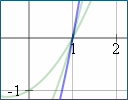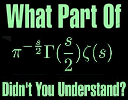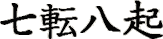Search IntMath
Close

By Murray Bourne, 18 May 2011

18 May 2011

1. Math tip: Finding a quadratic function from its graph
2. IntMath Poll: What stresses you?
3. Resource: Flat World Knowledge
4. Math tip: Understanding math formulas
5. Is there a place for invention in math?
6. Friday math movies
7. Final thought: perseverance

## 1. How to find the equation of a quadratic function from its graphA reader asked how to find the equation of a parabola from its graph. This is an important math skill, called modelling. How to find the equation of a quadratic function from its graph

## 2. IntMath Poll: What stresses you?

Add your vote to the current survey on any page in IntMath.com.

## 3. Resource: Flat World KnowledgeFlatworld Knowledge has an interesting approach. They offer "Premium open textbooks by top authors. Fully editable, Totally free." You can view the books at no cost, while there are pay options for printing or eBook versions (iPad, Kindle, etc). It's worth a look. Open source math books

(Only elementary algebra in the math section for now - more to come. They also have business, economics, and science books. Look for the "Read free now" button.)

## 4. Math tip: Understanding math formulas

Suitable for: Everyone - especially those with exams coming up.Many students struggle with the formulas in math. Here's some useful tips.

You may also enjoy the related article I mentioned last Newsletter:

How to learn math formulas

## 5. Is there a place for invention in math?

Suitable for: Everyone.Little kids invent things all the time. Should we let them (and their older siblings) invent math? Is there a place for invention in math?

## 6. Friday math movies

(a) Stephen Wolfram on Computing a theory of everythingThe developer of Wolfram|Alpha shares his views on how they've made huge improvements to normal search engines. Friday math movie: Stephen Wolfram on Computing a theory of everything

(b) Two DotsNow here's an interesting way to think of Euclidean geometry (points, lines and triangles). Friday math movie: Two Dots

## 7. Final thought – perseverance

It's very easy to get stressed while doing math. How often have you screamed "I give up!" when doing math homework?

The Japanese have a great saying that all math students should remember, especially when feeling frustrated:

Fall seven times, stand up eight. [Japanese proverb]

In Japanese, we say this as "Nana korobi yaoki" and write:The first character is nana (the number 7) and the 3rd character is ha (the number 8).

Until next time, enjoy whatever you learn.

### Comment Preview

HTML: You can use simple tags like <b>, <a href="...">, etc.

To enter math, you can can either:

1. Use simple calculator-like input in the following format (surround your math in backticks, or qq on tablet or phone):
a^2 = sqrt(b^2 + c^2)
(See more on ASCIIMath syntax); or
2. Use simple LaTeX in the following format. Surround your math with $$ and $$.
$$\int g dx = \sqrt{\frac{a}{b}}$$
(This is standard simple LaTeX.)

NOTE: You can mix both types of math entry in your comment.